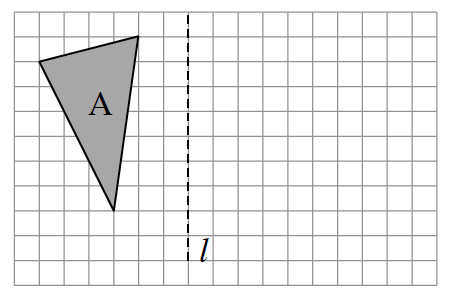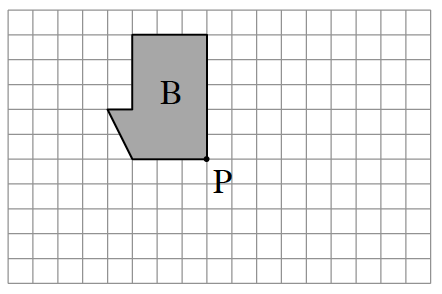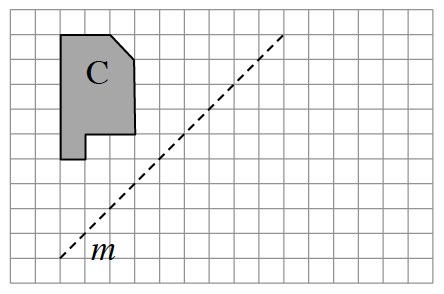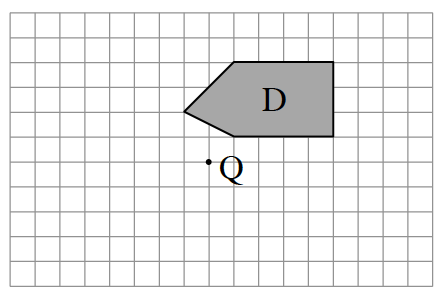Home > CC4 > Chapter 3 > Lesson 3.3.3 > Problem3-140

3-140.

Copy the diagrams below on graph paper. Then find the result when each indicated transformation is performed. 3-140 HW eTool (Desmos) Homework Help ✎

1. Reflect Figure A across line $l$.1. Rotate Figure B $90º$ clockwise $(\circlearrowright)$ about point P.1. Reflect Figure C across line $m$.1. Rotate Figure D $180º$ about point Q.The eTool below shows the transformations for all parts.
Click the link at right for the full version of the eTool: Int1 3-140 HW eTool.